# Noise Suppression Products/EMI Suppression Filters

Noise Suppression Basic Course Section 1
Chapter 1
Chapter 2
Chapter 3
Chapter 4
Chapter 5
Chapter 6
Chapter 6

## EMI suppression filters

### 6-3. Low-pass filters using LC

Low-pass filters are generally made from capacitors and inductors. Although creating filters from capacitors and inductors is a routine task for circuit designers, this section reviews the basic characteristics.

#### 6-3-1. Capacitors

##### (1) Bypass the noise current to ground

As shown in Fig. 1, a low-pass filter is formed by installing a capacitor parallel to the load.
Capacitor impedance by nature decreases as the frequency increases. This means that, the higher the frequency, the lower the voltage on the load. This is because, as shown in the figure, the noise current is bypassed by the capacitor and no longer flows to the load.

##### (2) Great for high impedance circuits

This noise will not be bypassed unless the impedance of the capacitor is relatively smaller than the output impedance and the impedance of the load. This means that it is easier for a capacitor to work if the surrounding circuit has a high impedance.
The impedance of the surrounding circuit is 50 ohms when measuring insertion loss. However, it is often not 50 ohms when a filter is used for noise suppression, so there is no particular fixed value. In order to estimate the noise reduction effect when a filter is actually used, it must be converted depending on the impedance of the surrounding circuit, based on the value measured with insertion loss.
This will be explained in Section 6-4. For now, let us assume that the impedance of the surrounding circuit is 50 ohms, to make it easier to understand the basic characteristics.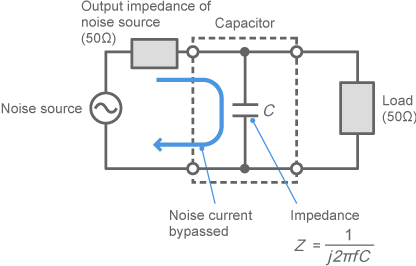Fig. 1 Low-pass filter made with a capacitor

#### 6-3-2. Basic characteristics of low-pass filters made with capacitors

##### (1) The higher the frequency, the greater the effect

When the logarithm of the frequency axis (horizontal axis) is plotted, the frequency characteristics of a low-pass filter made with a capacitor form a straight line with a slope of 20dB/dec. in the attenuation range, as shown in Fig. 2. This is because the impedance of the capacitor is inversely proportional to the frequency. If the frequency increases tenfold, the impedance of the capacitor will fall to one-tenth and the insertion loss will change by 20dB.
The "dec" (decade) mentioned above and elsewhere in this course means that the frequency has changed tenfold.

##### (2) The larger the electrostatic capacitance, the greater the effect

As shown in the figure, if the electrostatic capacitance of the capacitor is changed, the insertion loss curve undergoes parallel displacement. If the electrostatic capacitance of the capacitor changes tenfold, the insertion loss in the attenuation range likewise changes by 20dB. This is because it will be reduced to one-tenth, since the impedance of a capacitor is inversely proportional to the electrostatic capacitance.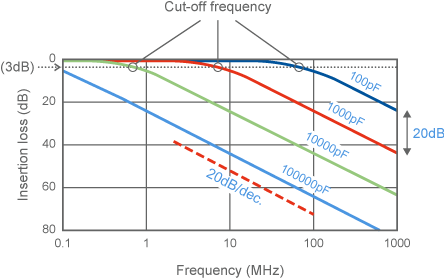Fig. 2 Basic characteristics of low-pass filters made with capacitors

##### (3) Cut-off frequency

In general, the frequency characteristics of a low-pass filter cling to 0dB in the low-frequency range (transmission range) , and demonstrate a significant insertion loss in the high-frequency range (attenuation range) . The frequency at which insertion loss becomes 3dB is used as the frequency to split these two ranges. This is called the cut-off frequency. As shown in Fig. 3, the cut-off frequency is roughly the lower limit frequency at which the filter exerts an effect.
If measured at 50 ohms, the cut-off frequency of a bypass capacitor will be the frequency at which the impedance of the capacitor is around 25 ohms.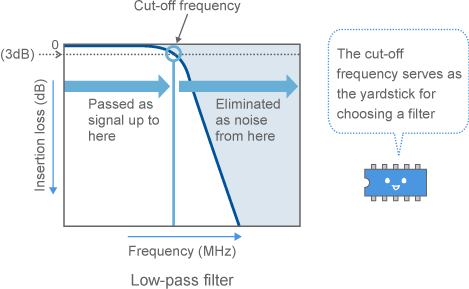Fig. 3 Cut-off frequency

#### 6-3-3. Inductors

##### (1) "Choke" noise currents

As shown in Fig. 7, inductors are installed parallel to the load.
Inductor impedance by nature increases as the frequency increases. This means that, the higher the frequency, the more difficult it is for noise currents to pass and, therefore, the lower the voltage on the load. Inductors used for this purpose are called choke coils, as they work to "choke" current in this manner.

##### (2) Great for low impedance circuits

An inductor will not be able to choke noise current unless the impedance of the inductor is relatively larger than the internal impedance of the signal source and the impedance of the load. This means that, in contrast with a capacitor, it is easier for an inductor to work when the surrounding circuit has a low impedance.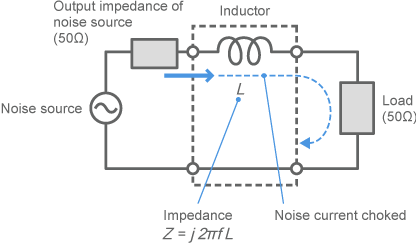Fig. 4 Low-pass filter made with an inductor

#### 6-3-4. Basic characteristics of low-pass filters made with inductors

##### (1) Same slope of 20dB/dec. as a capacitor

As shown in Fig. 5, the frequency characteristics of a low-pass filter made with an inductor form a straight line with a slope of 20dB/dec. in the attenuation range—just like with a capacitor. This is because the impedance of the inductor increases proportional to the frequency. If the frequency increases tenfold, the impedance will also increase tenfold and the insertion loss will change by 20dB.

##### (2) Result increases in proportion with inductance

As shown in the figure, if the inductance of the inductor is changed, the insertion loss curve undergoes parallel displacement. This is the same as with a capacitor.

##### (3) Cut-off frequency

If measured at 50 ohms, the cut-off frequency of an inductor will be the frequency at which the impedance of the inductor is around 100 ohms.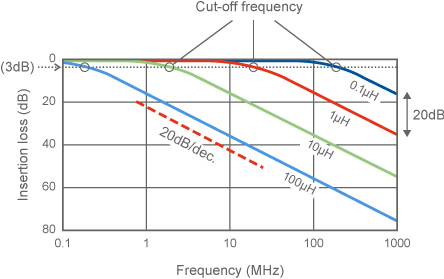Fig. 5 Basic characteristics of low-pass filters made with inductors

#### 6-3-5. Change in current when using capacitors and inductors

##### (1) The flow of noise becomes visible

How is a current bypassed or choked when using a capacitor or inductor? Fig. 6 and Fig. 7 show the results of using the magnetic near-field measurement system that was introduced in Chapter 3 to measure current distribution before and after using a filter.

##### (2) Observe noise flowing along the signal line

Fig. 6 shows the measurement conditions. The same measurement system that was used to measure the current standing wave in 3-3-4 is used here. As shown in Fig. 6 (b), a single signal line was run horizontally in the center of a 300mm × 100mm circuit board. The underside of the circuit board and the entire area of the surface other than the signal line are grounded. From the left of this signal line, a probe is used to measure the near magnetic field when a 33MHz digital signal is input, and the current is distributed. A digital IC is connected to the end of the signal line.
Grounded this way, the signal line can be thought of as a transmission line called an MSL. The width of the signal line has been adjusted so that the characteristic impedance is 50 ohms.
A store-bought measuring instrument called an EMI tester is used to measure the near magnetic field. In the figure, the measurement area measures 290m × 30mm, the measurement pitch is 5mm, and the observed frequency is 99MHz. 99MHz is the third harmonic of the 33MHz digital signal.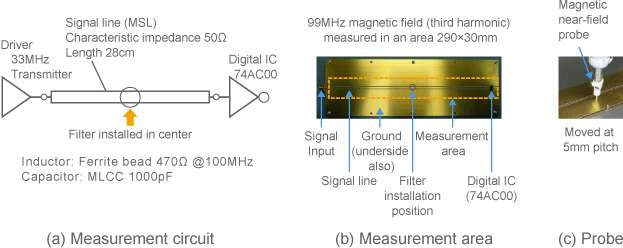Fig. 6 Near magnetic field measurement conditions

##### (3) Noise distribution with no filter

Changes to current distribution were then observed when a bypass capacitor and then an inductor were installed in the center of this signal line (150mm from the signal input). The measurement results are shown in Fig. 7.
Fig. 7 (a) is the same as the data shown in Fig. 19 (a) from 3-3-8, but with no filter. In the figure, noise enters from the left and, as it flows toward the terminal digital IC on the right edge, the signal gradually grows weaker. This is because a current standing wave is formed since the input impedance is high but the current is low at the digital IC on the right edge, and roughly one-half cycle of the standing wave is observed in this observational range.

##### (4) Observation of noise bypassed with a capacitor

Fig. 7 (b) shows what happens when a capacitor is used. A 1000pF MLCC is used between the signal line and ground.
The measurement results show that the current is strong on the left side and weak on the right side of the central filter. This can be interpreted as showing that noise entering from the left side is bypassed by the capacitor, and blocked from being output to the right side.
Using a capacitor in this way causes noise to be reflected strongly back to the noise source, so the current is stronger between the noise source and capacitor. Filters are therefore used as close to the noise source as possible, so that noise from this portion of the line is not emitted.

##### (5) Choke the noise current with an inductor

Fig. 7 (c) shows what happens when an inductor is used. A ferrite bead (470 ohms @100MHz) is inserted parallel to the signal line.
The measurement results show that the current is weaker on the left and right (both sides) of the central filter. This can be interpreted as showing that the effect of choking a current with an inductor extends even to the noise source.
Inductors also weaken the current on the side of the noise source. This makes them quite valuable in applications where there is no space near the noise source, and there is no choice but to install a part to suppress noise on the wiring. However, their ability to block noise is generally inferior to that of capacitors.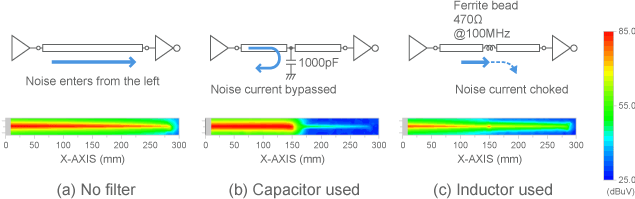Fig. 7 Near magnetic field results

Top of page